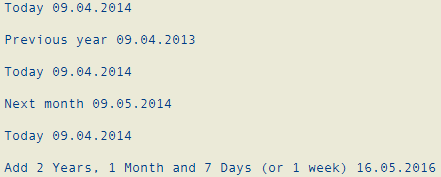﻿ ABAP Function to Calculate Date to Add Year Month Day to VariableSAP ABAP Programming and HANA Database Tutorials Development resources, articles, tutorials, code samples, tools and downloads for SAP HANA and ABAP, HANA Database, SQLScript, SAP UI5, Screen Personas, Web Dynpro, Workflow

# Add Year, Month or Day to Date Variable in ABAP using RP_CALC_DATE_IN_INTERVAL

In ABAP using RP_CALC_DATE_IN_INTERVAL function module, developers can calculate new date value by adding a time period of time expressed as years, months or days to a given input date parameter. Of course, ABAP developers can also substract date period to find a previous date just like adding to calculate later date values.

In this ABAP tutorial, I'll share sample ABAP codes using RP_CALC_DATE_IN_INTERVAL demonstrating how to calculate date by adding to current date in following sections. RP_CALC_DATE_IN_INTERVAL ABAP function module is very similar to SQL Server DateAdd() function.

Below ABAP code block calculates new date variable by substracting or adding years, months and days to a given date parameter. After new date is calculated using ABAP function module RP_CALC_DATE_IN_INTERVAL, I use WRITE function to display input and output date variables on the screen.
The first variable is the input date value which is today, the current date.
And the second date is calculated using RP_CALC_DATE_IN_INTERVAL function module in ABAP codes.

And the output of above ABAP program where source codes are shared is as follows:It is convenient to calculate date by using RP_CALC_DATE_IN_INTERVAL ABAP function module where developers are required to add or substract a time period which can be expressed in terms of years, months or days.

SAP HANA and ABAP